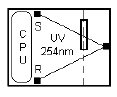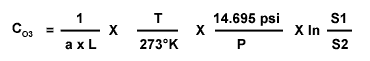OzoneLabOLA Theory of Ozone Module OperationHome > Products > Analyzers > Theory of Ozone Module Operation

The detection of ozone molecules is based on the absorption of 254 nm UV light due to an internal electronic resonance of the O3 molecule. The model OLA/DS uses a mercury lamp constructed so that a large majority of the light emitted is at the 254 nm wavelength.Light from the UV lamp shines through an absorption cell through which the sample gas being measured is passed. The ratio of the intensity of light passing through the gas is calculated from the UV light intensity measured by a sensor [S] and a reference measurement provided by the reference sensor [R], which does not pass through the gas, forms the ratio I/I0. This ratio forms the basis for the calculation of the ozone concentration.

The Beer-Lambert equation, shown below, calculates the concentration of ozone from the ratio of light intensities.Where:
 S1 Intensity of light passed through the sample S2 Intensity of light through ozone free gas sample a Absorption coefficient L Path length C03 Concentration of ozone T Sample temperature in degrees Kelvin P Pressure in pounds per square inch (absolute)

As can be seen the concentration of ozone depends on more than the intensity ratio. Temperature and pressure influence the density of the sample. The density changes the number of ozone molecules in the absorption cell, which impacts the amount of light, removed from the light beam. These effects are addressed by directly measuring temperature and pressure and including their actual values in the calculation. The absorption coefficient is a number that reflects the inherent ability of ozone gas to absorb 254 nm wavelength of the UV light. Lastly, the absorption path length determines how many molecules are present in the column of gas in the absorption cell.

The intensity of light is converted into a voltage by the detector/preamplifier. The voltage is converted into a number by a voltage-to-frequency (V/F) converter capable of 80,000 count resolution. The digitized signal, along with the other variables, is used by the CPU to compute the concentration of ozone using the above formula.